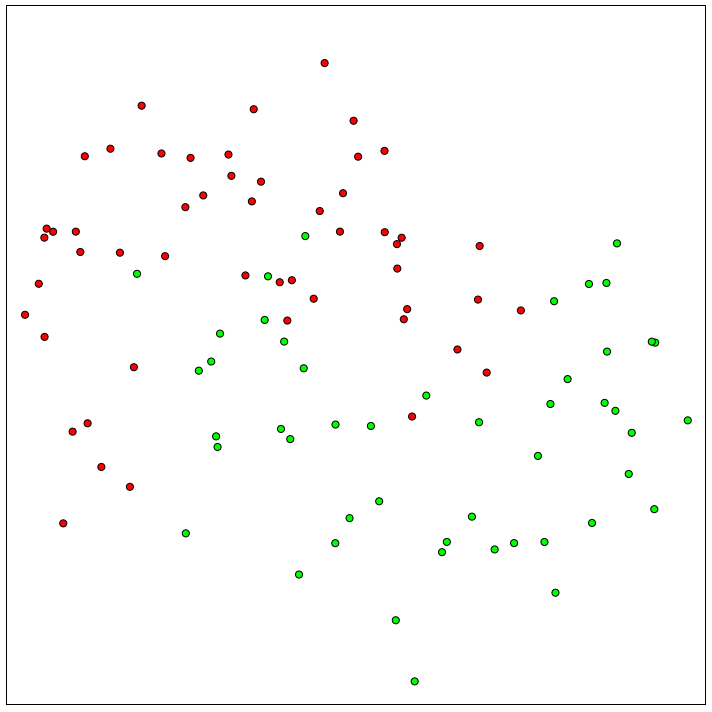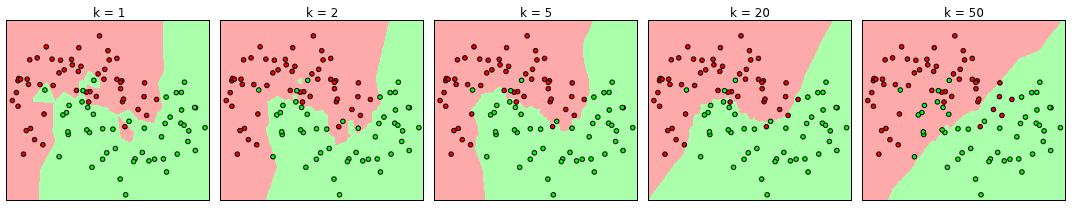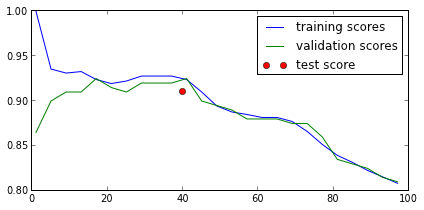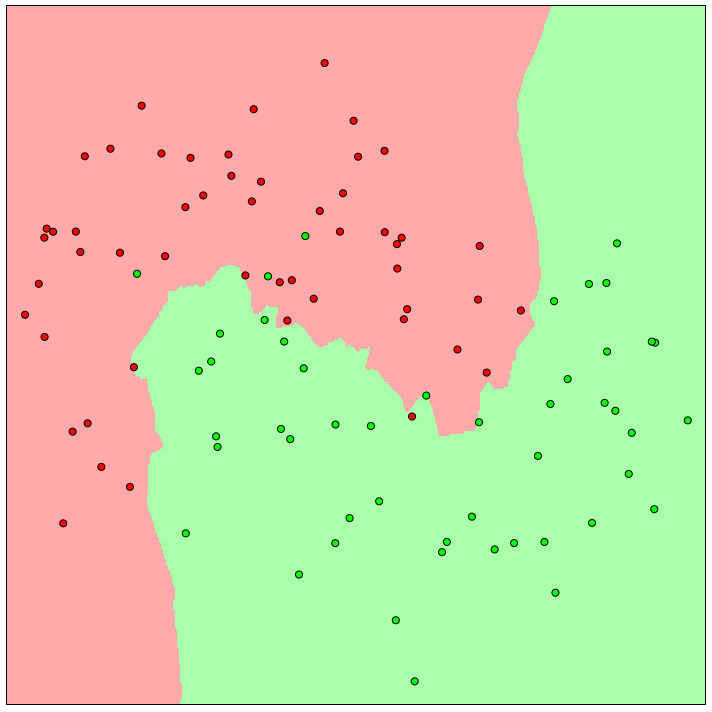In :
from sklearn import datasets
from sklearn.neighbors import KNeighborsClassifier
from matplotlib.colors import ListedColormap

In :
cmap_light = ListedColormap(['#FFAAAA', '#AAFFAA'])
cmap_bold = ListedColormap(['#FF0000', '#00FF00'])

h = .01  # step size in the mesh

x_min, x_max = X[:, 0].min() - .1, X[:, 0].max() + .1
y_min, y_max = X[:, 1].min() - .1, X[:, 1].max() + .1
xx, yy = np.meshgrid(np.arange(x_min, x_max, h),
np.arange(y_min, y_max, h))

#X, y = datasets.make_blobs(center_box=[-1, 1], random_state=2, n_samples=300, cluster_std=.2)
X, y = datasets.make_moons(noise=.3, n_samples=200, random_state=1)
plt.figsize(10, 10)

from sklearn.cross_validation import train_test_split
X_train, X_test, y_train, y_test = train_test_split(X, y, test_size=.5, random_state=0)
plt.scatter(X_train[:, 0], X_train[:, 1], c=y_train, cmap=cmap_bold, s=50)
plt.xlim(x_min, x_max)
plt.ylim(y_min, y_max)
plt.xticks(())
plt.yticks(())
plt.tight_layout()
plt.savefig("presentation/knn-pics/two_moons.pdf")In :
plt.figsize(15, 3)

n_neighbors = [1, 2, 5, 20, 50]
fig, axes = plt.subplots(1, len(n_neighbors))
for ax, k in zip(axes, n_neighbors):
clf = KNeighborsClassifier(n_neighbors=k)
clf.fit(X_train, y_train)

Z = clf.predict(np.c_[xx.ravel(), yy.ravel()])

# Put the result into a color plot
Z = Z.reshape(xx.shape)
ax.contourf(xx, yy, Z, cmap=cmap_light)

# Plot also the training points
ax.scatter(X_train[:, 0], X_train[:, 1], c=y_train, cmap=cmap_bold)
ax.set_xticks(())
ax.set_yticks(())
ax.set_xlim(x_min, x_max)
ax.set_ylim(y_min, y_max)
ax.set_title("k = %d" % k)
plt.tight_layout()
plt.savefig("presentation/knn-pics/two_moons_varying_k.pdf")In :
from sklearn.neighbors import KNeighborsClassifier
from sklearn.cross_validation import StratifiedKFold
train_scores = []
test_scores = []
ks = range(1, 100, 4)
for i in ks:
knn = KNeighborsClassifier(n_neighbors=i)

this_train = []
this_test = []
for train, test in StratifiedKFold(y, 10):
knn.fit(X[train], y[train])
this_train.append(knn.score(X[train], y[train]))
this_test.append(knn.score(X[test], y[test]))

train_scores.append(np.mean(this_train))
test_scores.append(np.mean(this_test))
plt.figsize(6, 3)
plt.plot(ks, train_scores, label="training scores")
plt.legend(loc="best")
plt.tight_layout()
plt.savefig("presentation/knn-pics/two_moons_cross_validation_1.pdf")
plt.plot(ks, test_scores, label="validation scores")
plt.legend(loc="best")
plt.savefig("presentation/knn-pics/two_moons_cross_validation_2.pdf")
best_k = 40
knn = KNeighborsClassifier(n_neighbors=best_k)
knn.fit(X, y)
X_, y_ = datasets.make_moons(noise=.3, n_samples=200, random_state=2)
plt.plot(best_k, knn.score(X_, y_), 'o', label="test score")
plt.legend(loc="best")
plt.savefig("presentation/knn-pics/two_moons_cross_validation_3.pdf")

/home/local/lamueller/checkout/scikit-learn/sklearn/neighbors/classification.py:131: NeighborsWarning: kneighbors: neighbor k+1 and neighbor k have the same distance: results will be dependent on data order.
neigh_dist, neigh_ind = self.kneighbors(X)
/home/local/lamueller/checkout/scikit-learn/sklearn/neighbors/classification.py:131: NeighborsWarning: kneighbors: neighbor k+1 and neighbor k have the same distance: results will be dependent on data order.
neigh_dist, neigh_ind = self.kneighbors(X)
/home/local/lamueller/checkout/scikit-learn/sklearn/neighbors/classification.py:131: NeighborsWarning: kneighbors: neighbor k+1 and neighbor k have the same distance: results will be dependent on data order.
neigh_dist, neigh_ind = self.kneighbors(X)
/home/local/lamueller/checkout/scikit-learn/sklearn/neighbors/classification.py:131: NeighborsWarning: kneighbors: neighbor k+1 and neighbor k have the same distance: results will be dependent on data order.
neigh_dist, neigh_ind = self.kneighbors(X)
/home/local/lamueller/checkout/scikit-learn/sklearn/neighbors/classification.py:131: NeighborsWarning: kneighbors: neighbor k+1 and neighbor k have the same distance: results will be dependent on data order.
neigh_dist, neigh_ind = self.kneighbors(X)
/home/local/lamueller/checkout/scikit-learn/sklearn/neighbors/classification.py:131: NeighborsWarning: kneighbors: neighbor k+1 and neighbor k have the same distance: results will be dependent on data order.
neigh_dist, neigh_ind = self.kneighbors(X)
/home/local/lamueller/checkout/scikit-learn/sklearn/neighbors/classification.py:131: NeighborsWarning: kneighbors: neighbor k+1 and neighbor k have the same distance: results will be dependent on data order.
neigh_dist, neigh_ind = self.kneighbors(X)
/home/local/lamueller/checkout/scikit-learn/sklearn/neighbors/classification.py:131: NeighborsWarning: kneighbors: neighbor k+1 and neighbor k have the same distance: results will be dependent on data order.
neigh_dist, neigh_ind = self.kneighbors(X)
/home/local/lamueller/checkout/scikit-learn/sklearn/neighbors/classification.py:131: NeighborsWarning: kneighbors: neighbor k+1 and neighbor k have the same distance: results will be dependent on data order.
neigh_dist, neigh_ind = self.kneighbors(X)
/home/local/lamueller/checkout/scikit-learn/sklearn/neighbors/classification.py:131: NeighborsWarning: kneighbors: neighbor k+1 and neighbor k have the same distance: results will be dependent on data order.
neigh_dist, neigh_ind = self.kneighbors(X)In :
plt.figsize(10, 10)
ax = plt.gca()
if True:
clf = KNeighborsClassifier(n_neighbors=5)
clf.fit(X_train, y_train)

Z = clf.predict(np.c_[xx.ravel(), yy.ravel()])

# Put the result into a color plot
Z = Z.reshape(xx.shape)
ax.contourf(xx, yy, Z, cmap=cmap_light)

# Plot also the training points
ax.scatter(X_train[:, 0], X_train[:, 1], c=y_train, cmap=cmap_bold, s=50)
ax.set_xticks(())
ax.set_yticks(())
ax.set_xlim(x_min, x_max)
ax.set_ylim(y_min, y_max)
#ax.set_title("k = %d" % 5)
plt.tight_layout()
plt.savefig("presentation/knn-pics/two_moons_k=5.pdf")In :


In [ ]: